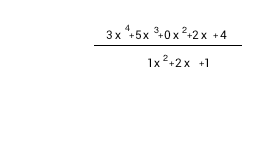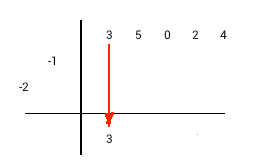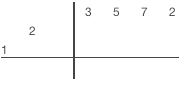# Synthetic division

A simple method of polynomial division.

Synthetic division is a method of Euclidean division of polynomials with a small number of writing and calculations. This calculator performs synthetic division of any polynomials. You may find the method description just below the calculator.#### Synthetic division

The polynomial coefficients, space separated, in order from higher term degree to lower
Divider polynomial coefficients, space separated, in order from higher term degree to lower
Result

Remainder

Synthetic division details

#### Synthetic division preparation

The synthetic division preparation by example: 3x4+5x3+2x+4 / x2+2x+1.

Preparation steps1) Negate divisor coefficients
2) Write dividend coefficients at the top (zero for missed terms).
3) Remove highest divisor coefficient.
4) Write remained divisor coefficients diagonally on the left

#### Synthetic division method for monic divisors

The synthetic division with monic divisor by example: 3x4+5x3+2x+4 / x2+2x+1.

Division algorithm for monic divisor1) Drop highest dividend coefficient in the first column of result row
2) Multiply divisor diagonal by the last column value of result row
3) Place multiplication result diagonally to the right from the last result column
4) Perform addition in the next column and write the sum in the same column of result row
5) Repeat steps 2-4 until you would go past the columns at the top row.
6) Sum values in any remaining columns and write the result in result row.
7) Separate result and remainder. A number of terms in the remainder equal a number of divisor terms minus one.
Example 1 (monic divisor)

#### Non-monic divisors

The synthetic division with non-monic divisor example: 3x3+5x2+7x+2 / 3x2-x-2.

Division algorithm for non-monic divisor1) Drop highest dividend coefficient in the first column of remainder row
2) Divide last column value in a remainder row by the first divisor coefficient, write the result in result row 3) Multiply divisor diagonal by the last column value of result row
4) Place multiplication result diagonally to the right from the last result column
5) Perform addition in the next column and write the sum in the same column of remainder row
6) Repeat steps 2-5 until you would go past the columns at the top row.
7) Sum values in any remaining columns and write the result in a remainder row.
8) Separate result and remainder.
Result coefficient will be in the last row. Remainder coefficients are in the previous row. A number of terms in the remainder equal to a number of divisor terms minus one.
Example 2 (non-monic divisor)
URL copied to clipboard

#### Similar calculators

PLANETCALC, Synthetic division